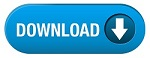Home » SSC » Model Questions for Scientific Assistant (IMD) Exam (Paper-2) PHYSICS – Set 42

# Model Questions for Scientific Assistant (IMD) Exam (Paper-2) PHYSICS – Set 42

## 1. Speed ‘v’ with which wave travels through a medium is given by

A. modulus of elasticity/density of medium
B. modulus of elasticity/”(density of medium)
C. Ö(modulus
of elasticity/density of medium)
D. v=d/t

## 2. Hooke’s law states that

A. extension is proportional to load when elastic limit is not exceeded
B. extension is inversely proportional to load when elastic limit is not
exceeded
C. extension is independent of load when elastic limit is not exceeded
D. load is dependent on extension

## 3. Dimensions of strain are

A. [L] B. [M] [L]-1 [T]-2
C. [L]-1
D. It’s a dimensionless quantity

A. undamped
B. free
C. damped
D. dependent

## 5. At ‘yield point’ of a copper wire

A. load hasn’t exceeded elastic limit yet; so, Hooke’s law applies
B. load has already exceeded elastic limit and material has become plastic
C. even plastic stage has passed and wire has snapped already
D. Like Brass and Bronze, Copper has no yield point

### 6. Stationary waves are also called

A. static waves
B. standing waves
C. progressive waves
D. All of above

## 7. When work done in moving a particle round a closed loop in a field is zero, forces in field are called

A. Zero forces
B. Non-Conservative forces
C. Conservative forces
D. Viscous forces

## 8. Substances that elongate considerably and undergo plastic deformation before they break are known as

A. brittle substances
B. breakable substances
C. ductile substances
D. elastic substances

A. 1 ND m²
B. 1 mm Hg
C. 1 bar
D. All of above

## 10. Velocity of sound waves through any material depends on

A. material’s density ‘d’ only
B. material’s density ‘d’ as well as its modulus of elasticity ‘E’
C. material’s modulus of elasticity ‘E’ only
D. neither material’s density ‘d’ nor its modulus of elasticity ‘E’

#